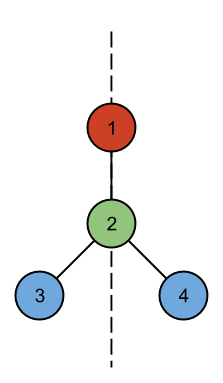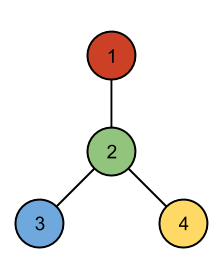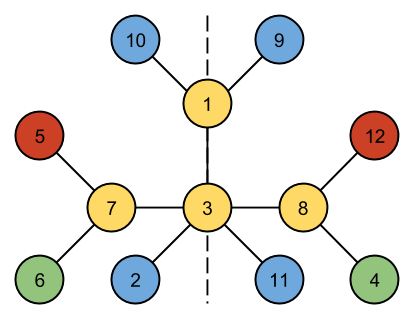시간 제한메모리 제한제출정답맞힌 사람정답 비율
5 초 512 MB139866.667%

## 문제

Given a vertex-colored tree with N nodes, can it be drawn in a 2D plane with a line of symmetry?

Formally, a tree is line-symmetric if each vertex can be assigned a location in the 2D plane such that:

• All locations are distinct.
• If vertex vi has color C and coordinates (xi, yi), there must also be a vertex vi' of color C located at (-xi, yi) -- Note if xi is 0, vi and vi' are the same vertex.
• For each edge (vi, vj), there must also exist an edge (vi', vj').
• If edges were represented by straight lines between their end vertices, no two edges would share any points except where adjacent edges touch at their endpoints.

## 입력

The first line of the input gives the number of test cases, T. T test cases follow.

Each test case starts with a line containing a single integer N, the number of vertices in the tree.

N lines then follow, each containing a single uppercase letter. The i-th line represents the color of the i-th node.

N-1 lines then follow, each line containing two integers i and j (1 ≤ i < jN). This denotes that the tree has an edge from the i-th vertex to the j-th vertex. The edges will describe a connected tree.

Limits

• 1 ≤ T ≤ 100.
• 2 ≤ N ≤ 12.

## 출력

For each test case, output one line containing "Case #x: y", where x is the case number (starting from 1) and y is "SYMMETRIC" if the tree is line-symmetric by the definition above or "NOT SYMMETRIC" if it isn't.

## 예제 입력 1

3
4
R
G
B
B
1 2
2 3
2 4
4
R
G
B
Y
1 2
2 3
2 4
12
Y
B
Y
G
R
G
Y
Y
B
B
B
R
1 3
1 9
1 10
2 3
3 7
3 8
3 11
4 8
5 7
6 7
8 12


## 예제 출력 1

Case #1: SYMMETRIC
Case #2: NOT SYMMETRIC
Case #3: SYMMETRIC


## 힌트

The first case can be drawn as follows:No arrangement of the second case has a line of symmetry:One way of drawing the third case with a symmetry line is as follows:## 채점 및 기타 정보

• 예제는 채점하지 않는다.